## The Bead Unbaffled - Totton Heffelfinger & Gary Flom

Abacus is a Latin word meaning sand tray. The word originates with the Arabic "abq", which means dust or fine sand. In Greek this would become abax or abakon which means table or tablet.

### Early Counting Boards and Tablets

Probably, the first device was the counting board. This appeared at various times in several places around the world. The earliest counting boards consisted of a tray made of sun dried clay or wood. A thin layer of sand would be spread evenly on the surface, and symbols would be drawn in the sand with a stick or ones finger. To start anew, one would simply shake the tray or even out the sand by hand.

Eventually, the use of sand was abandoned. Instead, pebbles were used, and placed in parallel grooves carved into stone counting boards. The oldest surviving counting board is the Salamis tablet, used by the Babylonians circa 300 B.C. It was discovered in 1846 on the island of Salamis. It is made of white marble and is in the National Museum of Epigraphy, Athens.

Later counting boards were made of various other materials. Besides the marble used by the Greeks, bronze was used by the Romans. As part of their primary education, young boys in both Greece and Rome learned at least some arithmetic using an abax or abacus. In fact, Plato suggested, "As much as is necessary for the purposes of war and household management and the work of government."

At some point, the Romans added additional grooves between each decimal position. So now, the grooves would signify 1s, 5s, 10s, 50s, 100s, 500s, 1000s, etc. This corresponded to the Roman numerals I, V, X, L, C, D, M. The Latin term for pebble is calculus. So, while calculus is considered higher mathematics, the term actually refers, literally, to the ancient counting boards and pebbles.

In the quest for an easily portable counting device, the Romans invented the hand abacus. This consisted of a metal plate with metal beads that ran in slots. The beads were held to the device by flanging on the back, but left loose enough to allow movement of the metal beads in the slots. The Roman hand abacus on display in the London Science Museum would fit in a modern shirt pocket. The bead arrangement is like the modern soroban (see later discussion), in that it has one bead in the relatively short upper slots, and four beads in the longer lower slots. There is a photograph of another Roman hand abacus at the Museo Nazionale Ramano at Piazzi delle Terme, Rome.

Some people believe that the Roman abacus, which pre-dates the Chinese suan-pan, was introduced into China early in the Christian era by trading merchants.

In the Middle ages, counting tables were quite common throughout Europe. In France, the counting pebbles were called jetons inspiring this little rhyme.

Les courtisans sont des jetons
Leur valeur dépend de leur place
Dans la faveur, des millions
Et des zeros dans la disgrâce

Translation

The courtiers are the counters
Their worth depends on their place
In favor, they're in the millions
And in the zeros when in disgrace

### The Framed Bead Abacus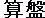Some of the first records of a device with counters that were strung on parallel rods have been found among relics of the Mayan civilization. The Aztec abacus, known as the nepohualtzitzin, dates back to the 10th century. The counting beads were made of maize strung along parallel wires or strings within a frame of wood. In addition, there was a bar across the frame that separated the counters into 3 above and 4 below the bar. This was consistent with the vigesimal (base 20) system thought to be used in ancient Aztec civilization as well as by the Basques in Europe. Each of the 3 counters above the bar represented 5. And each of the 4 counters below the bar represented 1. So a total of 19 could be represented in each column.

During the 11th century, the Chinese abacus, or suan pan, was invented. The suan pan is generally regarded as the earliest abacus with beads on rods. The Mandarin term suan pan means calculating plate. A suan pan has 2 beads above a middle divider called a beam (a.k.a. reckoning bar) and 5 beads below.

Use of the suan pan spread to Korea, and then to Japan during the latter part of the 15th century. The Japanese termed the abacus a soroban. Originally the soroban looked very much like its Chinese cousin having two beads above the reckoning bar and five beads below. Around 1850, it was modified to have only one bead above the reckoning bar while maintaining the five beads below. It was further changed by removing one lower bead in 1930. This one bead above and four beads below ( 1/4 ) arrangement remains as the present day Japanese soroban construction. In 1928, soroban examinations were established by the Japanese Chamber of Commerce and Industry. Over one million candidates had sat for the exams by 1959.

The Russians devised their own abacus, and call it a schoty. It was invented during the 17th century. The schoty has ten beads per rod , and no reckoning bar. Each bead counts as one unit. Usually the fifth and sixth beads are of a contrasting color to aid in counting. Many schoty have been found with various numbers of beads per column, including various numbers of beads per column on the same abacus.

Abaci are still in use today. They have been invaluable for many visually impaired individuals, as teaching number placement value and calculations can be done by feel. Merchants and bankers in various parts of the world still depend on an abacus for their day-to-day business. And as recently as 25 years ago (1979), the Chinese Abacus Association was founded. They established a graded examination in 1984, and started competitions in 1989. Areas without electricity, or inconsistent power, benefit from an abacus. And as an arithmetic teaching tool, it has been of immense value. In fact Forbes.com ranks the abacus as the second most important tool of all time. Many parts of the world teach abacus use starting as early as pre-kindergarten. It is felt by many that learning abacus strengthens the student's sense of number placement value and helps to further a better overall understanding of numbers. It's also a lot of fun.

Doubtlessly the Westerner, with his belief in the powers of mental arithmetic and the modern calculating machine, often mistrusts the efficiency of such a primitive looking instrument. However, his mistrust of the soroban is likely to be transformed into admiration when he gains some knowledge concerning it. For the soroban, which can perform in a fraction of time a difficult arithmetic calculation that the Westerner could do laboriously only by means of pencil and paper, possesses distinct advantages over mental and written arithmetic. In a competition in arithmetic problems, an ordinary Japanese tradesman with his soroban would easily outstrip a rapid and accurate Western accountant even with his adding machine. - Takashi Kojima, from his book, The Japanese Abacus, it's use and theory. Tokyo: Charles E. Tuttle, 1954

Every parent wants what's best for their children. There's no question that once the emphasis of the marketing of Soroban is moved from computation skills to the development of thinking skills, then it will be a no-brainer sell for parents. - George Sato, Better@Math~Better@Thinking, New York City, 2007

###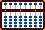ABACUS TECHNIQUES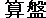The following techniques are Japanese and use a modern 1:4 bead Japanese soroban. I love Japanese soroban and it is the style of abacus I most often use. Collecting them has become a passion. Many soroban are beautifully crafted and wonderful to look at; especially the older ones. Some of the soroban in my collection have been signed by the craftsmen who made them and I love the way they work and feel. Some of them have been signed by their original owners. Each has its own history.

However, for some people the soroban may not be the instrument of choice. As already mentioned the Chinese have their own version of the abacus, a 2:5 bead suan pan. There are those who prefer to use a Chinese instrument because it has a larger frame and larger beads allowing for larger fingers. It really doesn't matter which instrument you use. The procedures are virtually the same for both and these methods are well suited to either instrument.

### The Japanese Soroban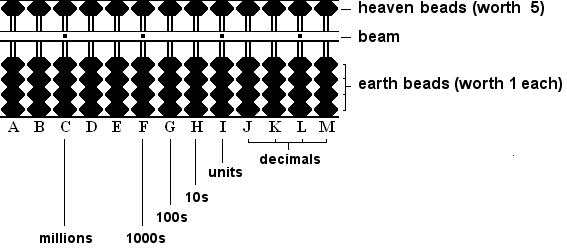Fig.1

A soroban is made up of a frame with vertical rods on which beads move up and down. Dividing the upper and lower portion of the soroban is a horizontal bar called a beam or reckoning bar.

### The Beam

On a modern-day soroban one bead sits above the beam and four beads sit below. The beads above the beam are often called heaven beads and each has a value of 5. The beads below are often called earth beads and each has a value of 1.

Along the length of the beam, you'll notice that every third rod is marked with a dot. These specially marked rods are called unit rods because any one of them can be designated to carry the unit number. While the soroban operator makes the final decision as to which rod will carry the unit number, it is common practice to choose a unit rod just to the right of center on the soroban.

The dots also serve as markers by which larger numbers can be quickly and efficiently recognized. For example in Fig.1 (above), rod I is the designated unit rod. For example, when given a number such as  23,456,789  an operator can quickly identify rod B as the 10 millions rod and go ahead and set the first number 2 on that rod. This ensures that all subsequent numbers will be set on their correct rods and that the unit number 9 will fall neatly on unit rod I.

### Setting Numbers on a Soroban

Use only the thumb and index fingers to manipulate beads on a soroban. The thumb moves the earth beads up toward the beam. The index finger moves everything else (all earth beads down away from the beam and all heaven beads up & down).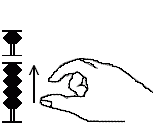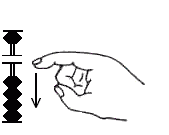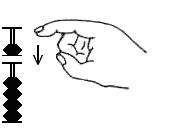Earth beads up (Fig.2) Earth beads down (Fig.3) Heaven beads down (Fig.4) Heaven beads up (Fig.5)

When setting numbers on the soroban the operator slides beads up or down so that beads touch the beam. Bringing up one earth bead so that it touches the beam gives a rod a value of 1. Three earth beads touching the beam give that rod a value of 3. To make a value of 5 clear all the earth beads and move one heaven bead down so that it touches the beam. Pinching together one heaven bead and two earth beads sets a value of 7 and so on.

In Fig.6 from left to right, the numbers on single rods show 1, 3, 5, 7 & 9. Designating rod F as the unit rod, the soroban on the right shows the number 42,386 on rods B, C, D, E and F. (Notice how the "2" in 42 thousand falls neatly on thousands rod C.)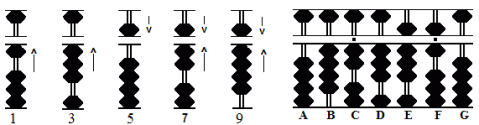Fig.6

### Clearing a Soroban

Calculations normally begin with an empty or cleared soroban. Place the soroban flat on the table, then tilt the frame toward you. Gravity pulls all the beads down. At this point only the earth beads have been cleared away from the beam. Place the soroban back onto the table and hold it with the left hand. Then, using the back of the right index finger, make a sweeping motion from left to right between the top of the beam and the bottom of the heaven beads (Fig.7). This forces the heaven beads up away from the beam. When none of the rods shows any value, this is what is known as a cleared frame.Fig.7

### Always Work from Left to Right

Fundamental to good soroban technique is the rule always work from left to right. This may seem a little odd at first but it's extremely important. It's one of the soroban's biggest advantages. It allows us to solve mathematical problems with great agility and speed, in part, because numbers are added and subtracted in exactly the same way we read and hear them.

To better understand this take the addition example 237 + 152 = 389 (see below). Working left to right set 237 onto the soroban. Now solve the problem: add 1 to the hundreds rod, 5 to the tens rod and finish by adding 2 to the units rod leaving 389.

It's the same for subtraction. This time take the example 187 - 125 = 62 (see below). Working left to right set 187 onto the soroban. Solve the problem: subtract 1 from the hundreds rod, 2 from the tens rod and complete the problem by subtracting 5 from the units rod leaving 62.

When using a soroban to solve problems of addition and subtraction, the process can often be quite straightforward and easy to understand. In each of the six examples below beads are either added or subtracted as needed.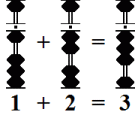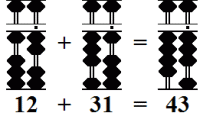Simple Subtraction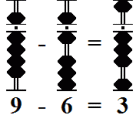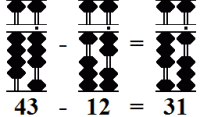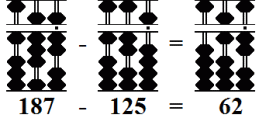But what happens when an operator is presented with a situation where rods don't contain enough beads to complete addition or subtraction problems in a simple, straightforward manner? This is where the real fun begins. In the next section we'll see how the use of complementary numbers and a process of mechanization allows an operator to add or subtract sets of numbers with lightning speed.

### A Process of Thoughtlessness

In competent hands, a soroban is a very powerful and efficient calculating tool. Much of its speed is attributed to the concept of mechanization. The idea is to minimize mental work as much as possible and to perform the task of adding and subtracting beads mechanically, without thought or hesitation. In a sense to develop a process of thoughtlessness. With this in mind, one technique employed by the operator is the use of complementary numbers with respect to 5 and 10.

• In the case of 5, the operator uses two groups of complementary numbers:
4 & 1 and 3 & 2.

• In the case of 10, the operator uses five groups of complementary numbers:
9 & 1,   8 & 2,   7 & 3,   6 & 4,   5 & 5.

With time and practice using complementary numbers becomes effortless and mechanical. Once these techniques are learned, a good operator has little difficulty in keeping up with (even surpassing) someone doing the same addition and subtraction work on an electronic calculator.

The following examples illustrate how complementary numbers are used to help solve problems of addition and subtraction. In all cases try not to think beforehand what the answer to a problem will be. Learn these simple techniques and and you'll be amazed at how quickly and easily correct answers materialize, even when problems contain large strings of numbers.

In addition, always subtract the complement.

#### Example 1:  Add   4 + 8 = 12

• Set 4 on rod B.
• Because rod B doesn't have 8 available, use the complementary number.

• The complementary number for 8 with respect to 10 is 2.
• Therefore subtract the complementary 2 from 4 on rod B and carry 1 to tens rod A.
• The answer 12 remains.  (Fig.8b)

4 + 8 = 12 becomes 4 - 2 + 10 = 12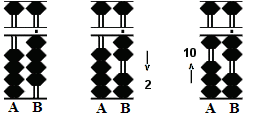Fig.8b
 Similar exercises: 4+9 4+7 4+6 3+9 3+8 3+7 2+9 2+8 1+9 9+9 9+8 9+7 9+6 8+9 8+8 8+7 7+9

#### Example 2:  Add  6 + 7 = 13

• Set 6 on rod B.
• Once again subtract the complement because rod B doesn't have the required beads.

• The complementary number for 7 with respect to 10 is 3.
• Therefore subtract the complementary 3 from 6 on rod B and carry 1 to tens rod A.
• This leaves the answer 13.  (Fig.9)

6 + 7 = 13  becomes 6 - 5 + 2 + 10 = 13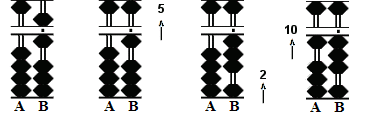Fig.9
 Similar exercises: 5+6 5+7 5+8 5+9 6+6 6+8 7+6 7+7 8+6

### Subtraction

In subtraction, always add the complement.

#### Example 1:  Subtract  11 - 7 = 4

• Set 11 on rods AB.
• Subtract 7.
• Since rod B only carries a value of 1 use the complement.

Please note: In subtraction the order of working the rods is different from that of addition.

• The complementary number for 7 with respect to 10 is 3.
• Begin by subtracting 1 from the tens rod on A,
• then add the complementary 3 to rod B to equal 4.  (Fig.10)

11 - 7 = 4 becomes 11 - 10 + 3 = 4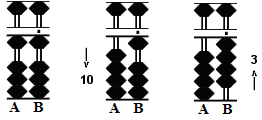Fig.10b
 Similar exercises: 10-6 10-7 10-8 10-9 11-8 11-9 12-8 12-9 13-9 15-9 15-8 15-7 15-6 16-9 16-8 16-7 17-9

#### Example 2:  Subtract  13 - 6 = 7

• Set 13 on rods AB.
• Subtract 6.
• Use the complement again.

• In this case, the complementary number for 6 with respect to 10 is 4.
• Begin by subtracting 1 from the tens rod on A,
• then add the complementary 4 to rod B to equal 7.  (Fig.11)

13 - 6 = 7 becomes 13 - 10 + 5 - 1 = 7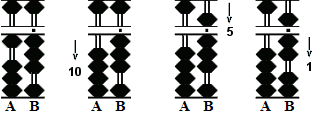Fig.11
 Similar exercises: 11-6 12-6 12-7 13-7 13-8 14-6 14-7 14-8 14-9

### The Order of the Rod

This is where students new to soroban can make mistakes. In each of the above examples the operation involves using two rods, a complementary number and a carry over from one rod to another. Notice the order of operation.

1. First subtract the complement from the rod on the right.
##### For Subtraction
1.  First subtract a bead from the rod on the left.
2.  Then add the complement to the rod on the right.

This is the most efficient order of operation. When attention is finished on one rod the operator moves on to the next. There is no back and forth between rods. This saves time.

### COMBINING FINGER MOVEMENTS

From the beginning it's important to start thinking about manipulating beads quickly and effectively. With this in mind try to combine finger movements.

In the last century, long before electronic calculators became commonplace, a governing body know as the Abacus Committee of the Japan Chamber of Commerce and Industry would regularly hold examinations  (75.7 kb PDF File) for individuals wishing to obtain a license to work as an abacus operator. These examinations began at the tenth grade level (the easiest) and worked up to the first grade level (most difficult). Those individuals who passed first, second or third grade examinations qualified for employment in government or business concerns as abacus operators.

During the examinations contestants were given lists of problems. The problems had to be solved within a given time frame. Of course accuracy was very important. But the best operators were also very fast, in part because they learned to combine finger movements wherever possible.

In some of the following examples I've put two combinations together in one problem. Any combination can always be used in conjunction with another or on its own. The nice thing about combination moves is that they don't really have to be committed to memory. Logically they all make sense and, more often than not, it just seems natural to use them.

Here are a few examples illustrating how operators  combine finger movements.

Example 1:  8 + 7 = 15

When students first begin to think about combining finger movements, I think it's this type that comes most naturally.

1. Using the thumb and index finger together, pinch the 5 bead and 3 earth beads onto rod C. This sets a  value of 8. (Two movements become one)

2. Combine finger movements. In a twisting movement, simultaneously use the index finger to subtract 3 earth beads from rod C while using the thumb to add a 10 bead onto rod B. (Two movements become one)Step 1 Step 2

By combining finger movements in Steps 1 & 2, the operator is able to complete the problem using only two finger movements instead of four.

 Similar Exercises:
 6+9 7+8 7+9 8+8 8+9 9+6 9+7 9+8 9+9

Example 2:
15 + 8 = 23

1. Simultaneously use the thumb and index finger together. In a twisting movement set the 5 bead on rod C and a 10 bead on rod B. This sets a  value of 15. (Two movements become one)

2. Once again combine the finger movements. Simultaneously use the thumb and index finger. In one movement add 3 beads with the thumb and subtract 5 with the index finger on rod C. (Two movements become one)

3. Use the thumb to move up the 10 bead on rod B. (One movement)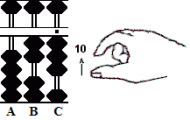Step 1 Step 2 Step 3

By combining finger movements in Steps 1 & 2, the operator is able to complete the problem using only three finger movements instead of five.

 Similar Exercises:
 15+6 15+7 15+9 6+6 6+7 6+8 7+6 7+7 8+6

Example 3:
9 + 3 = 12

1. Using the thumb and index finger together, pinch the 5 bead and 4 earth beads onto rod C. This sets a  value of 9. (Two movements become one)

2. Use the index finger to clear 2 beads from rod C. (One movement)

3. Combine the finger movements. Simultaneously use the index finger to subtract 5 from rod C while using the thumb to add a 10 bead on rod B . (Two movement become one)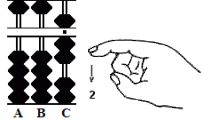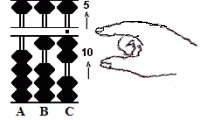Step 1 Step 2 Step 3

By combining finger movements in Steps 1 & 3, the operator is able to complete the problem using only three finger movements instead of five.

 Similar Exercises
 6+4 7+3 7+4 8+2 8+3 8+4 9+1 9+2 9+4

Example 4:
14 - 9 = 5

1. Use the thumb for both movements. Set a 10 bead on rod B and 4 earth beads on rod C. (Two movements)

2. Use the index finger to subtract the 10 bead from rod B. (One movement)

3. Combine the finger movements. In one fluid motion use the index finger to add the 5 bead to rod C and clear the 4 earth beads below. (Two movements become one)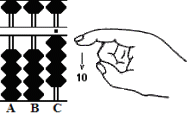Step 1 Step 2 Step 3

Using this combination in Step 3, the operator is able to complete the problem using only four finger movements instead of five.

 Similar Exercises
 11-6 12-6 12-7 13-6 13-7 13-8 14-6 14-7 14-8

Example 5:
11 - 7 = 4  (This is a combination I've not seen illustrated. Even so, it's one I like to use.)

1. Use the thumb for both movements. Set a 10 bead on rod B and 1 earth bead on rod C. (Two movements)

2. Combine the finger movements. Simultaneously use the index finger to subtract a 10 bead on rod B while using the thumb to add 3 earth beads onto rod C. (Two movements become one)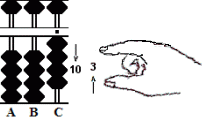Step 1 Step 2

Using this combination in Step 2, the operator is able to complete the problem using only three finger movements instead of four.

 Similar Exercises
 10-6 10-7 10-8 10-9 11-8 11-9 12-8 12-9 13-9

### Simplifying the Process of Mechanization

This is another look at the process of mechanization. Many operators find that this method helps to simplify adding and subtracting numbers onto a soroban. For those new to abacus work, this chapter may be better studied at a later time.

### Simple, Fast and Efficient

In part a soroban is a recording device that helps keep track of place value. Consequently the process of adding and subtracting numbers is greatly simplified because, at most, only two rods will ever come into play at any given time. This is another of the soroban's greatest strengths. For example, when given an addition problem that involves adding a number such as seven hundred forty two thousand, six hundred and fifty-three, the soroban allows us to break the problem down into six manageable, easy to remember segments. Each segment is then solved on a rod by rod basis. It's that simple. Seven, four, two, six, five, three are quickly added to their respective rods. This, in conjunction with working left to right, makes solving problems extremely fast and very efficient.

Of the four principal arithmetic disciplines most commonly done on the soroban; addition, subtraction, multiplication and division, it is really addition and subtraction that are the most important. They form the foundation for all soroban work.

###ADDITION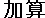Example: 135 + 321 = 456

Step 1: With rod H acting as the unit rod, set 135 on rods FGH. (Fig.12)Fig.12 ```Step 1 A B C D E F G H I . . .   0 0 0 0 0 1 3 5 0 ```

Step 2: Add 3 to hundreds rod F.
Step 3: Add 2 to tens rod G.
Step 4 and the answer: Add 1 to units rod H leaving the answer 456 on rods FGH. (Fig.13)Fig.13 ```Step 2 A B C D E F G H I . . .   0 0 0 0 0 1 3 5 0 + 3 Step 2 0 0 0 0 0 4 3 5 0 + 2 Step 3 0 0 0 0 0 4 5 5 0 + 1 Step 4 0 0 0 0 0 4 5 6 0```

The above is pretty straightforward. This next example gets a little tricky. When using the complementary numbers there are several 'carry-overs' from right to left.

Example: 456 + 567 = 1,023

Step 1: Set 456 on rods FGH using H as the unit. (Fig.14)Fig.14 ```Step 1 A B C D E F G H I . . .   0 0 0 0 0 4 5 6 0 ```

Step 2: Add 5 to hundreds rod F leaving 956 on rods FGH. (Fig.15)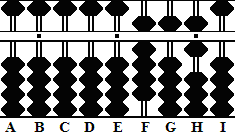Fig.15 ```Step 2 A B C D E F G H I . . .   0 0 0 0 0 4 5 6 0 + 5 Step 2 0 0 0 0 0 9 5 6 0 ```

Step 3: Add 6 to tens rod G. Use complimentary numbers. Subtract the complementary 4, then....

3a: Carry 1 to hundreds rod F. No beads. Subtract the complementary 9, then....

3b: Carry 1 over to thousands rod E leaving 1016 on rods EFGH. (Fig.16)Fig.16 Using complementary numbers, the  process of adding 6 to rod G becomes: ` Step 3` ``` A B C D E F G H I . . .   0 0 0 0 0 9 5 6 0 - 4 Step 3 0 0 0 0 0 9 1 6 0 - 9 Step 3a 0 0 0 0 0 0 1 6 0 + 1 Step 3b 0 0 0 0 1 0 1 6 0```

Step 4:
Add 7 to units rod H. Use complimentary numbers. Subtract the complementary 3 then....

4a and the answer: Carry 1 to tens rod G. This leaves the answer 1023 on rods EFGH. (Fig. 17)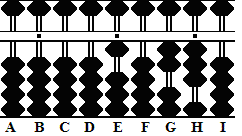Fig.17 Using complementary numbers, the  process of adding 7 to rod H becomes: ``` Step 4 A B C D E F G H I . . .   0 0 0 0 1 0 1 6 0 - 3 Step 4 0 0 0 0 1 0 1 3 0 + 1 Step 4a 0 0 0 0 1 0 2 3 0```

It is important to emphasize at this point that one should not fall into old habits. Many people will know that in this last step, for example, when adding 7 + 1016 the answer will be 1023. It doesn't take much skill to know this. Nevertheless, the idea here is to keep mental work to a minimum. Work using complementary numbers and allow the soroban to do its job. This is what makes the soroban such a powerful tool.

###SUBTRACTION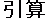Example: 4321 - 3456 = 865

Step 1: Set 4321 on rods EFGH with rod H acting as the unit.Fig.18 ```Step 1 A B C D E F G H I . . .   0 0 0 0 4 3 2 1 0 ```

Step 2: Subtract 3 from thousands rod E leaving 1321 on rods EFGH. (Fig.19)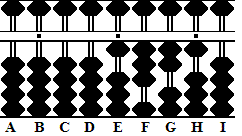Fig.19 ```Step 2 A B C D E F G H I . . .   0 0 0 0 4 3 2 1 0 - 3 Step 2 0 0 0 0 1 3 2 1 0 ```

Step 3: Subtract 4 from hundreds rods F. Use the complement. Subtract 1 from thousands rod E, then....

3a: Add the complement 6 to rod F leaving 921 on rods FGH. (Fig.20)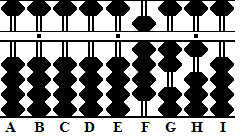Fig.20 ```Step 3 A B C D E F G H I . . .   0 0 0 0 1 3 2 1 0 - 1 Step 3 0 0 0 0 0 3 2 1 0 + 6 Step 3a 0 0 0 0 0 9 2 1 0 ```

Step 4: Subtract 5 from tens rod G. Use complimentary numbers. Subtract 1 from hundreds rod F, then....

4a: Add the complement 5 to rod G leaving 871 on rods FGH. (Fig.21)Fig.21 ```Step 4 A B C D E F G H I . . .   0 0 0 0 0 9 2 1 0 - 1 Step 4 0 0 0 0 0 8 2 1 0 + 5 Step 4a 0 0 0 0 0 8 7 1 0 ```

Step 5: Subtract 6 from units rod H. Use the complement. Subtract 1 from tens rod G, then....

5a and the answer: Add the complement 4 to rod H leaving the answer 865 on rods FGH. (Fig.22)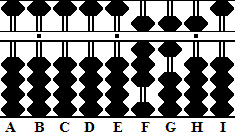Fig.22 ```Step 5 A B C D E F G H I . . .   0 0 0 0 0 8 7 1 0 - 1 Step 5 0 0 0 0 0 8 6 1 0 + 4 Step 5a 0 0 0 0 0 8 6 5 0 ```

 Further Examples: Subtraction

###MULTIPLICATION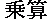While there are other techniques used to solve problems of multiplication on the soroban, the method described here is the one most commonly suggested by the Abacus Committee. In order to solve problems of multiplication using this technique one must know the multiplication tables up to 9 x 9 = 81. In the following examples I will use standard terminology. For example in the problem 6 x 3 = 18,  6 is the multiplicand, 3 is the multiplier and 18 is the product.

When setting up problems of multiplication on the soroban it is customary to set the multiplicand in the central part of the soroban with the multiplier set to the left leaving two empty rods in between. Always add the product immediately to the right of the multiplicand. In the case of a multiplicand consisting of two or more numbers: when the operator is finished using the first part of the multiplicand that number is then cleared from the soroban and the operation continues using the next part of the multiplicand. (See the examples below for clarification.)

Many soroban experts do not even bother to set the multiplier onto the soroban at all. Instead, they prefer to save a little time and set only the multiplicand.

Predetermine the Unit Rod

For problems where multipliers and multiplicands begin with whole numbers: In order to get the unit number of a product to fall neatly on a predetermined unit rod, place your finger on the chosen rod. Move your finger to the left counting off one rod for every digit in the multiplier plus one for every digit in the multiplicand. Set the first number in the multiplicand on that rod.

 For more detailed instructions on predetermining the unit rod see: Unit Rod

Example: 34 x 7 = 238

In this example choose rod H to be the unit rod. There is one number in the multiplier and two in the multiplicand. Count off three rods to the left ending up at rod E. Set the up the problem so that first number in the multiplicand falls on rod E. Now the unit number in the product will fall neatly on rod H.

Step 1: Set the multiplicand 34 on rods EF and the multiplier 7 on B. (Fig.23)Fig.23 ```Step 1 A B C D E F G H I . . .   0 7 0 0 3 4 0 0 0 ```

Step 2: Multiply the 4 on F by 7 on B. Add the product 28 immediately to the right of the multiplicand on rods GH.

2a: Having finished with this part of the multiplicand (the 4 on F) clear it from the soroban. This leaves 3 on rod E and the partial product 28 on rods GH. (Fig.24)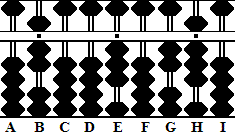Fig.24 ```Step 2 A B C D E F G H I . . .   0 7 0 0 3 4 0 0 0 + 2 8 Step 2 0 7 0 0 3 4 2 8 0 clear (-4) Step 2a 0 7 0 0 3 0 2 8 0 ```

Step 3: Multiply the 3 on E by 7 on B. Add the product 21immediately to the right of the multiplicand on rods FG.

3a and the answer: Clear the 3 on E from the soroban leaving the answer 238 on rods FGH. (Fig.25)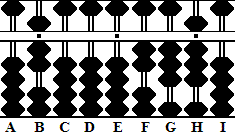Fig.25 ```Step 3 A B C D E F G H I . . .   0 7 0 0 3 0 2 8 0 + 2 1 Step 3 0 7 0 0 3 2 3 8 0 clear (-3) Step 3a 0 7 0 0 0 2 3 8 0 ```

It is a good idea to think of a product as having of at least two digits. For example 3 x 4 = 12 and 3 x 1 = 03. It helps in placing products on their correct rods.

Example: 2.3 x 17 = 39.1

In this example the multiplier has two whole numbers and the multiplicand has one for a total of three whole numbers. Choose rod I as the unit and count off three rods to the left. Set the fist number in the multiplicand on rod F.

Step 1: Set the multiplicand 23 on rods FG. Set the multiplier 17 on BC. (Fig.26)Fig.26 ```Step 1 A B C D E F G H I J K . . .     0 1 7 0 0 2 3 0 0 0 0 ```

Step 2: Multiply the 3 on G by 1 on B. Add the product 03 on rods HI.

2a: Next, multiply the 3 on G by 7 on C and add the product 21 on rods IJ.

2b: Clear the 3 on G. This leaves 2 on F and the partial product 51 on rods IJ. (Fig.27)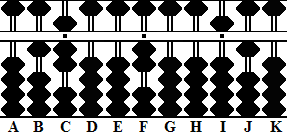Fig.27 ```Step 2 A B C D E F G H I J K . . .     0 1 7 0 0 2 3 0 0 0 0 + 0 3 Step 2 0 1 7 0 0 2 3 0 3 0 0 + 2 1 Step 2a 0 1 7 0 0 2 3 0 5 1 0 clear (-3) Step 2b 0 1 7 0 0 2 0 0 5 1 0 ```

Step 3: Multiply the 2 on F by 1 on B and add the product 02 on rods GH.

3a: Next, multiply the 2 on F by 7 on C and add the product 14 on rods HI.

3b and the answer: Clear the 2 on F leaving the answer 39.1 on rods HIJ. Notice how the unit number in the product has fallen on unit rod I and the first decimal number on rod J. (Fig.28)Fig.28 ```Step 3 A B C D E F G H I J K . . .     0 1 7 0 0 2 0 0 5 1 0 + 0 2 Step 3 0 1 7 0 0 2 0 2 5 1 0 + 1 4 Step 3a 0 1 7 0 0 2 0 3 9 1 0 clear (-2) Step 3b 0 1 7 0 0 0 0 3 9 1 0 ```

This standard technique for solving problems of multiplication is very powerful in part because it creates patterns that are easy to follow. For more on this see Patterns.

 Further Examples: Multiplication

###DIVISION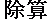As is the case with multiplication, there are different techniques that can be used to solve problems of division on the soroban. Although the method described below is often referred to as the 'modern method' it's not really all that new. It had been in use in Japan for many years before becoming widely popular around 1930 after improvements to the technique were made. This is the way division is most often done today. In the following examples I will use standard terminology. For example in the problem 6 � 3 = 2,  6 is the dividend, 3 is the divisor and 2 is the quotient.

When setting up problems of division on the soroban, the dividend is set on the right and the divisor is set on the left. The Abacus Committee suggests leaving 4 unused rods in between the two numbers. It's on these unused rods where the quotient answer is formed.

Often soroban experts do not bother to set the divisor. Instead, they prefer to save a little time and set only the dividend.

Division is done by dividing one number in the divisor into one or possibly two numbers of the dividend at a time. The operator multiplies after each division step and subtracts the product. The next part of the dividend is then tacked onto the remainder and the process continues. It is much like doing it with a pencil and paper.

### Division Revision

Easy Division Revision: In the event that the quotient is too high or too low, it is the ease with which the operator can revise an answer that makes the soroban such a powerful tool. Doing division work on a soroban allows the operator to make an estimate as to what a quotient might be, go a head and do the work then quickly make an adjustment if need be. (See the Division Revision section below.)

### Rules for Placing the First Quotient Number

Rule I) Where the digits in a divisor are less than (or equal to) the corresponding digits of the dividend, begin by placing the quotient two rods to the left of the dividend. In Fig.29, the divisor 4 is smaller than the dividend 8. The quotient begins two rods to the left of the dividend.two rods to the left. (Fig.29) one rod to the left. (Fig.30)

Rule II) Where the digits in the divisor are greater than the corresponding digits of the dividend, begin by placing the first number in the quotient one rod to the left of the dividend. In Fig.30 the divisor 4 is larger than the dividend 2. The quotient begins one rod to the left of the dividend.

### Predetermine the Unit Rod

For problems where divisors and dividends begin with whole numbers: The process of predetermining the unit rod is very much the same as it is for multiplication in that it involves counting digits and rods. However, it is slightly different and a little more involved. In division, the operator chooses a unit rod and then counts left the number of digits in the dividend. From that point, the operator counts back again to the right the number of digits plus two in the divisor. The first number in the dividend is set on that rod.

 For more detailed instructions on predetermining the unit rod see: Unit Rod

Example: 951 ÷ 3 = 317

In this example, the dividend has three whole numbers. Choose rod F as the unit and count three rods to the left. The divisor has one whole number so count one plus two back to the right. Set the first number of the dividend on rod F.

Step 1: Set the dividend 951 on rods FGH and the divisor 3 on A. (Fig.31)Fig.31 ```Step 1 A B C D E F G H I J K . . .     3 0 0 0 0 9 5 1 0 0 0 ```

Step 2: The divisor (3 on A) is smaller than the dividend (9 on F). Therefore apply "Rule I" and set the first number in the quotient two rods to the left on D. Divide 3 on A into 9 on F and set the quotient 3 on rod D.

2a: Multiply the quotient 3 by 3 on A and subtract the product 9 from rod F. This leaves the partial quotient 3 on D and the remainder of the dividend 51 on rods GH. (Fig. 32)Fig.32 ```Step 2 A B C D E F G H I J K . . .     3 0 0 0 0 9 5 1 0 0 0 (3) Step 2 - 9 Step 2a 3 0 0 3 0 0 5 1 0 0 0 ```

Step 3: Divide 3 on A into 5 on G. Once again the divisor is smaller than the dividend so follow "Rule1". Set the quotient 1 on rod E.

3a: Multiply the quotient 1 by 3 on A and subtract the product 3 from 5 on rod G. This leaves the partial quotient 31 on DE and the remainder of the dividend 21 on rods GH. (Fig.33)Fig.33 ```Step 3 A B C D E F G H I J K . . .     3 0 0 3 0 0 5 1 0 0 0 (1) Step 3 - 3 Step 3a 3 0 0 3 1 0 2 1 0 0 0 ```

Step 4: Divide 3 on A into 21 on GH and set the quotient 7 on rod F.

4a and the answer: Multiply the quotient 7 by 3 on A and subtract the product 21 from rods GH leaving the answer 317 on rods DEF. (Fig.34)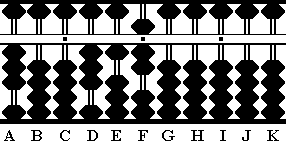Fig.34 ```Step 4 A B C D E F G H I J K . . .     3 0 0 3 1 0 2 1 0 0 0 (7) Step 4 - 2 1 Step 4a 3 0 0 3 1 7 0 0 0 0 0 ```

Example: 356 ÷ 25 = 14.24

In this example, the dividend has three whole numbers. Choose F as the unit rod and count three to the left. The divisor has two whole numbers so count two plus two back to the right. Set the first number of the dividend on rod G.

Step 1: Set the dividend 356 on GHI and the divisor 25 on rods AB. (Fig.35)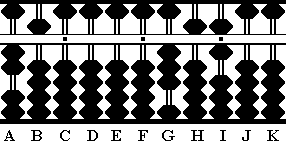Fig.35 ```Step 1 A B C D E F G H I J K . . .     2 5 0 0 0 0 3 5 6 0 0 ```

Step 2: Because the first two digits in the divisor are smaller than those of the dividend apply "Rule I" and begin placing the quotient two rods to the left on rod E. Divide 2 on rod A into the 3 on G and set the quotient 1 on rod E.

2a: Multiply the quotient 1 by 2 on A and subtract the product 2 from 3 on rod G.

2b: Multiply the quotient 1 by 5 on B and subtract the product 5 from 15 on rods GH. This leaves the partial quotient 1 on rod E and the remainder of the dividend 106 on rods GHI. (Fig.36)Fig.36 ```Step 2 A B C D E F G H I J K . . .     2 5 0 0 0 0 3 5 6 0 0 (1) Step 2 - 2 Step 2a 2 5 0 0 1 0 1 5 6 0 0 - 5 Step 2b 2 5 0 0 1 0 1 0 6 0 0 ```

Step 3: Divide 2 on A into 10 on GH. At first glance, it looks like the answer should be 5. However, in order to continue working the problem there must be a remainder. Instead, use the quotient 4. Set 4 on rod F.

3a: Multiply the quotient 4 by 2 on A and subtract the product 8 from 10 on rods GH.

3b: Multiply the quotient 4 by 5 on B and subtract the product 20 from rods HI. This leaves the partial quotient 14 on rods EF and the remainder 6 on rod I. (Fig.37)Fig.37 ```Step 3 A B C D E F G H I J K . . .     2 5 0 0 1 0 1 0 6 0 0 (4) Step 3 - 8 Step 3a 2 5 0 0 1 4 0 2 6 0 0 - 2 0 Step 3b 2 5 0 0 1 4 0 0 6 0 0 ```

In order to continue, borrow a zero from rod J. This yields 60 on rods IJ and creates the first decimal number in the quotient.

Step 4: Divide 2 on A into 6 on rod I. It looks like the answer should be 3. But once again, there must be enough of a remainder to continue working the problem. Instead, use the quotient 2. Follow "Rule 1" and set 2 on rod G.

4a: Multiply the quotient 2 by 2 on A and subtract the product 4 from 6 on rod I.

4b: Multiply the quotient 2 by 5 on B and subtract the product 10 from rods IJ. This leaves the partial quotient 14.2 on rods EFG and the remainder of the dividend 10 on rods IJ. (Fig.38)Fig.38 ```Step 4 A B C D E F G H I J K . . .     2 5 0 0 1 4 0 0 6 0 0 (2) Step 4 - 4 Step 4a 2 5 0 0 1 4 2 0 2 0 0 - 1 0 Step 4b 2 5 0 0 1 4 2 0 1 0 0 ```

Once again borrow a zero, this time from rod K. This yields 100 on rods IJK.

Step 5: Divide 2 on A into 10 on rods IJ. Again make sure there is enough of a remainder to continue working. Choose the quotient 4. Set 4 on rod H.

5a: Multiply the quotient 4 by 2 on A and subtract the product 8 from 10 on rods IJ.

5b and the answer: Multiply the quotient 4 by 5 on B and subtract the product 20 from rods JK leaving 1424 on rods EFGH. Because rod F is the designated unit rod the answer reads 14.24. (Fig.39)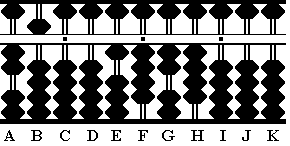Fig.39 ```Step 5 A B C D E F G H I J K . . .     2 5 0 0 1 4 2 0 1 0 0 (4) Step 5 - 8 Step 5a 2 5 0 0 1 4 2 4 0 2 0 - 2 0 Step 5b 2 5 0 0 1 4 2 4 0 0 0```
 Further Examples: Division

###DIVISION REVISION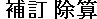In problems of division, coming to the correct answer can sometimes involve a little trial and error. The division problem shown below is a case in point. In this type of problem making a judgment as to what the exact quotient will be can be difficult. Estimation is often the best course of action. It's the ease with which an operator can revise an incorrect answer that makes the soroban such a superior tool for solving problems of division.

Example: 0.14 ÷ 1.6 = 0.0875

Choose D as the unit rod. In this example, because there are no whole numbers in the dividend there is no need to count to the left. The divisor has one whole number. Starting on rod D, count one plus two to the right. Set the first number of the dividend on rod G.

Step 1: Set the dividend 14 on rods GH and divisor 16 on rods AB. (Fig.40)Fig.40 ```Step 1 A B C D E F G H I J K . . .   .   1 6 0 0 0 0 1 4 0 0 0 ```

Step 2: In this problem, the first two digits in the divisor are larger than those of the dividend. Apply "Rule II". Set the first number in the quotient one rod to the left of the dividend, in this case on rod F. Think of the problem as dividing 16 on AB into 140 on GHI. Now estimate the quotient. It seems reasonable to estimate a quotient of 7. Set 7 next to the dividend on rod F.

2a: Multiply the quotient 7 by 1 on A and subtract the product 7 from 14 on rods GH.

2b: Multiply the quotient 7 by 6 on B and subtract the product 42 from rods HI.

With 28 remaining on rods HI, the estimated quotient of 7 was too small.

2c & 2d: Revise both the quotient and the dividend. Add 1 to the quotient on rod F to make it 8. Subtract a further 16 from rods HI. This leaves the partial quotient 0.08 on CDEF and the remainder 12 on HI. (Fig.41)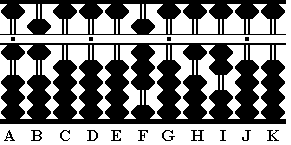Fig.41 ```Step 2 A B C D E F G H I J K . . .   .   1 6 0 0 0 0 1 4 0 0 0 (7) Step 2 - 7 Step 2a 1 6 0 0 0 7 0 7 0 0 0 - 4 2 Step 2b 1 6 0 0 0 7 0 2 8 0 0 plus 1 Revise(2c) - 1 6 Revise(2d) 1 6 0 0 0 8 0 1 2 0 0```

Step 3:
Borrow a zero from rod J and think of the problem as dividing 16 on AB into 120 on HIJ. Estimate a quotient of 8. Set 8 on rod G.

3a: Multiply the quotient 8 by 1 on A and subtract the product 8 from 12 on rods HI.

3b: Multiply the quotient 8 by 6 on B and subtract the product 48 from rods IJ.

In order to continue there must be at least 48 on rods IJ. There's only 40. The estimated quotient of 8 was too large.

3c & 3d: Revise both the quotient and the dividend. Subtract 1 from 8 on G. Add 1 to 4 on rod I.

3e & 3f: Continue with the problem but this time use the revised quotient of 7. Multiply 7 on G by 6 on B and subtract 42 from rods IJ. This leaves the partial quotient 0.087 on rods CDEFG and the remainder, 8, on rod J. (Fig.42)Fig.42 ```Step 3 A B C D E F G H I J K . . .   .   1 6 0 0 0 8 0 1 2 0 0 (8) Step 3 - 8 Step 3a 1 6 0 0 0 8 8 0 4 0 0 - ? ? Step 3b minus 1 Revise(3c) + 1 Revise(3d) 1 6 0 0 0 8 7 0 5 0 0 (7) Step 3e - 4 2 Step 3f 1 6 0 0 0 8 7 0 0 8 0 ```

Step 4:
Borrow a zero from rod K and think of the problem as dividing 16 on AB into 80 on JK. Estimate a quotient of 5. Set 5 on rod H.

4a: Multiply the quotient 5 by 1 on A and subtract the product 5 from 8 on rod J.

4b and the answer: Multiply the quotient 5 by 6 on B and subtract the product 30 from rods JK leaving 875 on rods FGH. With rod D acting as the unit, the answer reads 0.0875 on rods CDEFGH. (Fig.43)Fig.43 ```Step 4 A B C D E F G H I J K . . .   .   1 6 0 0 0 8 7 0 0 8 0 (5) Step 4 - 5 Step 4a 1 6 0 0 0 8 7 5 0 3 0 - 3 0 Step 4b 1 6 0 0 0 8 7 5 0 0 0```
 Further Examples: Revision

###NEGATIVE NUMBERS FROM SUBTRACTIONIn the following examples, negative numbers are a result of subtracting a larger number from a smaller one. Because a negative sign can not be placed on the soroban, the operation of subtracting larger numbers from smaller ones is performed on the soroban by means of complementary numbers.

### Complementary Numbers at a Glance

In order to see negative numbers on a soroban, it is necessary to be able to recognize complementary numbers quickly. From left to right the rods below show the values 3, 42 and 286.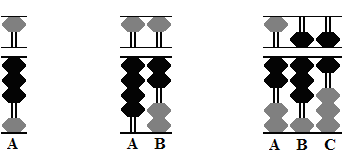Fig. 44 Fig. 45 Fig.46

Notice the grey beads. They form the basis for the complementary number. In order to find the true complementary number for each of the above add plus 1 to the total value of grey beads.

In Fig. 44, the grey beads add up to 6. Plus 1 equals 7, the complement of 3 with respect to 10.

In Fig.45, the grey beads add up to 57. Plus 1 equals 58, the complement of 42 with respect to 100.

In Fig.46, the grey beads add up to 713. Plus 1 equals 714, the complement of 286 with respect to 1,000.

 Rule i) Always add plus 1 to the last value of the complementary number to find the true complement.

PROBLEM: After making purchases totaling \$86.32 a customer gives a shopkeeper \$100.00. What is the amount of change due back to the customer?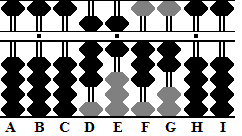Fig.47 SOLUTION: After placing the total \$86.32 onto the soroban the shopkeeper reads the complementary number. The grey beads total \$13.67. Plus 1 equals \$13.68 which is the amount of change owed to the customer. (Fig.47)

### Subtraction and the Negative Answer

Example: 13 - 78 = -65

Step 1: Set 13 on rods DE with E acting as the unit rod. (Fig.48)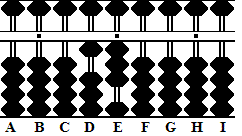Fig.48 ```Step 1 A B C D E F G H I . . .   0 0 0 1 3 0 0 0 0```

Step 2: Subtract 78 from 13. As it stands this is not possible and this tells us we are going to have a negative result. On a soroban the way to solve this problem is to borrow. To give us something to borrow set 1 on hundreds column C. This leaves 113 on rods CDE. (Fig.49)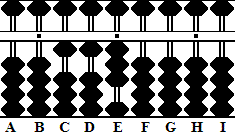Fig.49 ``` Step 2 A B C D E F G H I . . .   0 0 0 1 3 0 0 0 0 + (1) Step 2, borrow 100 0 0 1 1 3 0 0 0 0 ```

Except for practice purposes it is not really necessary to set the 1 on rod C as shown in Fig.49. It is enough to do it mentally.

Step 3 and the answer: With 113 on CDE there is enough to borrow and complete the operation. Subtract 78 from 113 leaving 35 on DE. The negative answer appears in the form of a complementary number as seen on the grey beads. Add plus 1 to the complementary number and the final answer is -65. (Fig.50)Fig.50 ```Step 3 A B C D E F G H I . . .   0 0 1 1 3 0 0 0 0 - 7 8 Step 3 0 0 0 3 5 0 0 0 0 ```

 Rule ii) If the number being subtracted is too large, borrow from the rods on the left and continue the operation as if doing a normal subtraction problem. Rule iii) Reading the complementary result yields the negative answer.

Example: Add 182 to the result in the above equation. [-65 + 182 = 117]

 step 1: The process of adding 182 to negative 65 is very easy. Simply add 182 to the 35 already set on rods DE. This equals 217. 1a and the answer: Subtract the 100 borrowed in Step 2 of the previous example (see above). This leaves the answer 117 on rods CDE, which is the answer. ```step 1 A B C D E F G H I . . .   0 0 0 3 5 0 0 0 0 + 1 8 2 Step 1 0 0 2 1 7 0 0 0 0 - (1) Step 1a, subtract 100 0 0 1 1 7 0 0 0 0```
 Rule iv) Give back the amount borrowed if subsequent operations yield a positive answer.

Example: 19 - 72 - 7846 = -7,899

Step 1: Set 19 on DE with E acting as the unit. (Fig.51)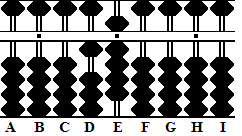Fig.51 ```Step 1 A B C D E F G H I . . .   0 0 0 1 9 0 0 0 0 ```

Step 2: In order to subtract 72 from 19 borrow 1 from the hundreds column on rod C leaving 119 on CDE

2a: Subtract 72 from 119 leaving 47 on rods DE. Interim, this leaves the complementary number 53 on rods DE. (Fig.52)Fig.52 ```Step 2 A B C D E F G H I . . . 0 0 0 1 9 0 0 0 0 + (1) Step 2, borrow 100 0 0 1 1 9 0 0 0 0 - 7 2 Step 2a 0 0 0 4 7 0 0 0 0 ```

In Step 2 (see above), the operation required borrowing 100 from rod D. In this next step it will be necessary to borrow again but borrowing a further 10,000 would bring the total to 10100. That would be wrong. Instead, borrow a further 9900 bringing the total amount borrowed to 10,000.

Step 3: In order to subtract 7846 from 47 on rods DE borrow 9900. This leaves 9947 on rods BCDE. (Fig.53)Fig.53 ```Step 3 A B C D E F G H I . . .   0 0 0 4 7 0 0 0 0 +(9 9) Step 3, borrow 9900 0 9 9 4 7 0 0 0 0 ```

 Rule v) If the operation requires borrowing again, only borrow 9s from adjacent rods. Remember that all complementary numbers on the soroban result from powers of 10. (10, 100, 1000 and so on.)

Step 4 and the answer: Subtract 7846 from 9947 leaving 2101 on rods BCDE. Once again, the negative answer appears in the form of a complementary number. Add plus 1 to the complementary number and the answer is -7899. (Fig.54)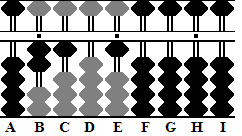Fig.54 ```Step 4 A B C D E F G H I . . . 0 9 9 4 7 0 0 0 0 - 7 8 4 6 Step 4 0 2 1 0 1 0 0 0 0 ```

Example: Subtract a further 31 from the result in the above equation.
[- 7899 - 31 = -7930]

Step 1 and the answer: Subtract 31 from 2101 leaving 2070 on rods BCDE. The complementary number shows 7920. Because rod E does not have a value add plus 1 to the complement on rod D instead. This leaves the complementary answer -7930. (Fig.55)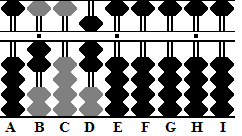Fig.55 ```Step 1 A B C D E F G H I . . .   0 2 1 0 1 0 0 0 0 - 3 1 Step 1 0 2 0 7 0 0 0 0 0 ```
 Rule vi) When adding plus 1 to the last digit of the complementary number add only to the last rod that has a value.

A small tutorial on how to multiply Negative numbers:
Multiplying Negative numbers

###CARING FOR YOUR ABACUS

In some cases, when you first use your abacus, it may be apparent that the frame is somewhat warped. By pressing down on each of the corners individually (when the abacus is lying in working position on a flat surface) you may determine that a corner, or its diagonally opposite corner, needs to be built up. An effective method to do this is by gluing small cut out "L" shaped pieces from a 3 x 5 card on the back of the frame at a needed corner. You can "titrate" this by gluing these one at a time on top of each other to the needed correction, checking for wobble after each piece is affixed. (Here's how I fixed one of the soroban I own. - TPH)

If you find that your abacus wants to slide on your table, putting a small stick on rubber foot on the underside of the frame at each corner is helpful. These are the same feet that are used under a table lamp to keep it from sliding. Use the small, approximately 1 cm, stick on rubber feet.

To clean the beads, rods, and frame, use a soft lint free rag dampened with a mild soap solution.

To keep the beads moving freely, the rods must be kept clean. If the rods are bamboo or rattan, treat occasionally with furniture polish. A good way to apply it, is by spraying the polish on a rag, then wiping the rods with it. Then buff with a soft dry rag. With regular use of the abacus and occasional polish of the rods, the bead movement will become quite silky.

If the rods are metal, then you may first have to remove some surface changes. This can be done with gentle scraping using the tip of a sharp blade. To obtain a smooth bead action on the rod, you can apply a thin coating of a teflon based lubricant, wiping the excess with a dry cloth.

You can also treat the frame and beads with furniture polish after cleaning them. It is a good idea to do this prior to storing an abacus if it will not be used for awhile. And whenever the wood seems dry or loses its luster.

To minimize dust accumulation, store them standing on end in a covered or enclosed area. Keep them in a low humidity and temperature controlled environment. Basically, treat them like your slide rules and other collectibles.

###ABACUS DIVERSIONS

These are a couple of exercises that are easy to remember.

"Crazy Eights"

• Add 98765432 , nine times , to get 888888888 .

"Repeated Number Sums"

• Add 123456789 , nine times , to get 1111111101 .
• Then , add 123456789 nine more times to 1111111101 and obtain 2222222202 .
• Adding 123456789 nine more times to that result will yield 3333333303 , and so on.
 The general formula for the above is : 123456789 times (9 times n) = nnnnnnnn0n.

You can also subtract 123456789 nine times from one of these "repeated number" sums to get back to the prior "repeated number" sum.

"Fibonacci Numbers"

1, 1, 2, 3, 5, 8, 13, 21, 34, 55, etc.

 General recursion formula: F(n) + F(n+1) = F(n+2) F(1) = 1 , F(2) = 1
• Choose a rod towards the left and set 1 . (call this "L#" ) .

• Choose a rod towards the right and set 1 . (call this "R#" ) .

2 on left
1 on right

2 on left
3 on right

5 on left
3 on right

5 on left
8 on right

And so on....

Can also work these in reverse, by back and forth subtraction, to get back to 1s.

### References

GENERAL ABACUS HISTORY AND INFORMATION

Books

Pullan, J.M.
The History of the Abacus
London: Books That Matter, 1968. pgs. 21, 25 & 30.

Moon, Parry.
The Abacus: Its history; its design; its possibilities in the modern world
New York: Gordon and Breach Science, 1971. pgs. 16 - 17.

Gullberg, Jan.
Mathematics From the Birth of Numbers
New York, London: W.W.Norton & Company, 1997.
ISBN : 0-393-04002-X
pgs 168 -170

Websites

Fernandes, Luis. "Introduction", The Abacus, the Art of Calculating with Beads
http://www.ee.ryerson.ca:8080/~elf/abacus/  (Updated: Jul 16 2004)

The Abacus: A History
E. A. Young
http://fenris.net/~lizyoung/abacus.html  (Revised September, 2004)

Wikipedia: http://en.wikipedia.org/wiki/Abacus

The League of Japan Abacus Associations
http://www.syuzan.net/english/index.html

SOROBAN TECHNIQUES

Books

Kojima, Takashi.
The Japanese Abacus: Its Use and Theory
Tokyo: Charles E. Tuttle, 1954.

Kojima, Takashi.
Advanced Abacus: Japanese Theory and Practice
Tokyo: Charles E. Tuttle, 1963.

The Japanese Chamber of Commerce & Industry.
Soroban, the Japanese abacus it's use and practice
Tokyo: Charles E. Tuttle, 1967.

Tani, Yukio
The Magic Calculator, the way of the abacus

Kato, Professor Fukutaro.
SOROBAN pelo Método Moderno (SOROBAN by the Modern Method)
Brazil: Brazilian Shuzan Cultural Association, (not dated - but looks to be circa 1969)

Martinez, Beluva Sulliuent.
Soroban in America
Tokyo: The league of Soroban Education of Japan Inc. (not dated - but looks to be circa 1980)

Websites

Bernazzani, Dave.
The Soroban Abacus HandBook.pdf ( 281 kb)
Rev.1.05 - March 2, 2005

Members of the Soroban / Abacus newsgroup
https://groups.io/g/sorobanabacus

http://totton.idirect.com/soroban/

TITLE PAGE PHOTOGRAPH

GRAPHIC IMAGES